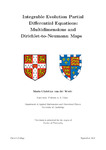## Integrable Evolution Partial Differential Equations: Multidimensions and Dirichlet-to-Neumann Maps

##### Authors
Van Der Weele, Maria Christina
##### DOIThesis
##### Abstract

This thesis is about integrable Partial Differential Equations (PDEs). Here "integrable" means that the given PDE or system of PDEs can be written as the compatibility condition of a set of linear equations, the so-called Lax pair, and can be solved via a method related to the Inverse Scattering Transform. Although integrable systems are quite rare, they play an extremely important role in physical applications, including the theory of water waves and optics. The thesis focuses on two main topics:

A. Solving multidimensional integrable PDEs

The modern history of integrable systems begins in the late 1960’s when Gardner et al. solved the initial value problem of the Korteweg-de Vries (KdV) equation by what was later called the Inverse Scattering Transform. For some years the KdV equation with its striking properties was thought to be a unique case, until Zakharov and Shabat showed that the Nonlinear Schrödinger (NLS) equation can also be solved using the same methodology. The KdV and NLS equations are integrable evolution equations in 1+1 dimensions (i.e., 1 spatial and 1 temporal dimension). There also exist integrable generalisations of these equations to 2+1 dimensions, such as the Kadomtsev-Petviashvili (KP) and Davey-Stewartson (DS) equations. One of the current topics of interest concerns generalisations to more than 2 spatial dimensions. The fact that integrable equations in 3 and 4 spatial dimensions do exist was proven in 2006 by A. S. Fokas, who explicitly constructed integrable equations in 4+2 dimensions. The associated initial value problem, where the functions to be determined are specified at t₁ = t₂= 0, can be solved by means of a nonlocal d-bar problem. Here we treat the multidimensional generalisations of the DS system and of the three-wave interaction equations. Starting from these equations in 2+1 dimensions (x, y and t), we construct their generalisations in 4+2 dimensions by complexifying each of these variables. We analyse these nonlinear equations in 4+2 dimensions, using a variety of techniques including nonlinear Fourier transforms, Green’s functions, the dressing method, and the direct linearising method. Moreover, in the linear limit of the DS system we show how this analysis can be reduced to 3+1 dimensions, i.e., with only 1 time variable. A separate section is dedicated to the construction of explicit solutions, including graphical representations, of the three-wave interaction equations in 4+2 dimensions.

B. Determining the Dirichlet-to-Neumann map for evolution PDEs on the half-line with time-periodic boundary conditions

For a well-posed boundary value problem, a certain number of boundary values must be prescribed as boundary conditions, while the rest of the boundary values are unknown. The task of determining the unknown boundary values in terms of the prescribed ones is called the computation of the "generalised Dirichlet-to-Neumann map". In the second part of this thesis, we elaborate on a new approach for finding the Dirichlet-to-Neumann map in the large time limit for evolution PDEs on the half-line, for the physically significant case of time-periodic boundary conditions. The new method is illustrated both for linear PDEs, including the heat equation, the convection-diffusion equation and the linearised KdV equation, and for integrable nonlinear PDEs, in particular for the focusing NLS equation. It is shown that the time-dependent part of the Lax pair is instrumental in yielding, via an elegant algebraic calculation, the large t asymptotics of the periodic unknown boundary values in terms of the prescribed periodic boundary data. This method is based on earlier work by Lenells and Fokas (2015), in which the NLS equation was treated via a more complicated approach.

2021-09-01